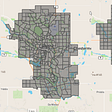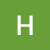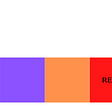# Solving Popular Algorithms: Replace “?”s

`let getRandomChar = (prev, nxt) => {    let alphaArr =   ['a', 'b', 'c', 'd', 'e', 'f', 'g', 'h', 'i',        'j', 'k', 'l', 'm', 'n', 'o', 'p', 'q', 'r', 's', 't', 'u', 'v', 'w', 'x', 'y', 'z']        let randomChar = alphaArr[Math.floor(Math.random() *   alphaArr.length)]    while (randomChar === prev || randomChar === nxt) {        randomChar = alphaArr[Math.floor(Math.random() * alphaArr.length)]    }        return randomChar}`
`var modifyString = function(s) {        let splitS = s.split("")        for (let i = 0; i < splitS.length; i++) {        if (splitS[i] === '?' && i > 0) {            let prev = splitS[i-1]            let nxt = splitS[i+1]            let newChar = getRandomChar(prev, nxt)             splitS.splice(i, 1, newChar)                              } else if (splitS[i] === '?') {            let nxt = splitS[i+1]            let newChar = getRandomChar("1", nxt)            splitS.splice(i, 1, newChar)         } else if (splitS[i] === '?' && !splitS[i+1]) {            let prev = splitS[i-1]            let newChar = getRandomChar(prev, "1")            splitS.splice(i, 1, newChar)        }    }        return splitS.join("")    };`

--

--

--

## More from HopeGiometti

Love podcasts or audiobooks? Learn on the go with our new app.

## Two Ways to Immediately Improve Your Python Code## Get bugs fixed faster with the right description## Azure Database for PostgreSQL — Flexible Server and Features## Master .NET Interactive with F### Leetcode — Design Add and Search Words Data Structure## Range Sum Query 2D — Immutable